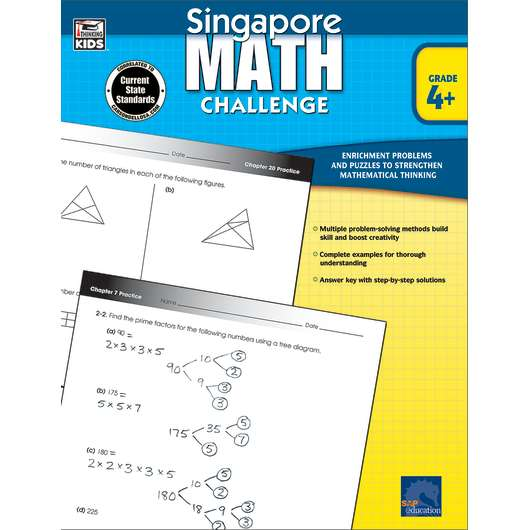•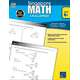•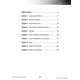•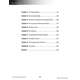•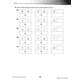•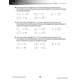# Singapore Math Challenge, Grades 4+

Product Number: FS-704281
CA\$22.48
Get ready to take the Math Challenge! Singapore Math Challenge will provide fourth grade students with skill-building practic...
Quantity

##### Common Core

Get ready to take the Math Challenge! Singapore Math Challenge will provide fourth grade students with skill-building practice based on the leading math program in the world, Singapore Math! Common Core Standards accelerate math expectations for all students, creating a need for challenging supplementary math practice. Singapore Math Challenge is the ideal solution, with problems, puzzles, and brainteasers that strengthen mathematical thinking. Step-by-step strategies are clearly explained for solving problems at varied levels of difficulty. A complete, worked solution is also provided for each problem. Singapore Math Challenge includes the tools and practice needed to provide a strong mathematical foundation and ongoing success for your students. The Common Core State Standards cite Singapore math standards as worldwide benchmarks for excellence in mathematics.
FS-704281